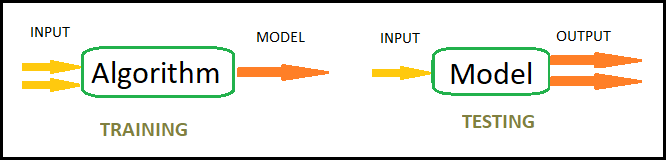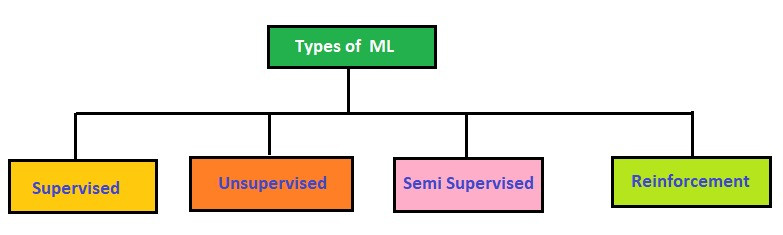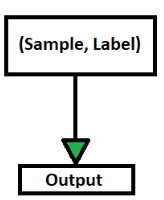Search

# Supervised Machine Learning Algorithms - A Sneak Peek

Machine learning is enabling computers to do tasks that have only been done by people, until now. From self- driving cars to recognizing speech and translating them, effective web search to understanding the human genome, machine learning is creating a storm in the field of Artificial Intelligence(AI) by helping software predict the unpredictable real world.

So, What is learning for a machine?

A machine is said to be learning from the large amount of data that has been fed to it and making predictions based on those data. It is basically to train the computer with the help of past data to predict future data.

Those predictions could be as simple as finding out whether the animal in a photo is a cat or a dog, to something like recognizing speech accurately to generate captions for a website or to run a video or music on YouTube.

There are so many algorithms that are out there to make a machine learn. The data is fed to these algorithms to create a model and with that model, data predictions are made.In this blog, I will talk about:

• Types of Machine Learning

• What is Supervised Learning?

• How Supervised Learning Works

• Types of Supervised Machine Learning Algorithms

• Applications of Supervised Learning

## Types of Machine Learning:

There are 4 types of machine learning as shown in the diagram.Let’s concentrate only on Supervised learning in this blog as the majority of practical machine learning uses supervised learning.

## What is Supervised Learning?

Supervised Learning is a very powerful tool to classify and process data in machine learning. In Supervised learning, the machine is trained using data which is "labeled". Labeled dataset is one which has both input and output parameters.

A supervised learning algorithm learns from labeled training data and helps us to predict outcomes for unforeseen data. We create a model using training data and when the machine is provided with a new set of examples(data) the model’s supervised learning algorithm analyses the training data(set of training examples) and predicts an outcome for the new set of data.With supervised learning, each piece of data that is passed to the model during training is a pair that consists of the entire object (Sample) along with the corresponding output value(Label) as shown in the figure.

So, essentially with supervised learning, the model is learning how to create a mapping from given inputs to particular outputs based on its learning from labelled training data.

## How Supervised Learning works?

For instance, let’s say we have different kinds of fruits and each fruit is tagged with a label. So here, the machine learns that an apple looks like this, a mango looks like this etc.So, once the training is done, the machine is fed with new data or test data. Here the machine is fed with an image of mango and the machine predicts that there is a 96% probability that the image is that of a mango.## Types of Supervised Machine Learning Algorithms

Supervised learning can again be divided in to:

1. Regression - Output variable is continuous in nature

2. Classification - Output variable is categorical in nature

## Regression:

A regression problem is when the output variable is a real or continuous value, such as “salary” or “weight”.

For example: Which of the following is a regression task?

• Predicting weight of a person

• Predicting gender of a person

• Predicting whether stock price of a company will increase tomorrow

• Predicting whether a document is related to ML or not?

Solution : Predicting weight of a person (because it is a real value, predicting gender is categorical, whether stock price will increase is discrete-yes/no answer, predicting whether a document is related to ML or not is again discrete- a yes/no answer).

Types of Regression Models:

• Linear regression - Simple linear regression is a statistical method that allows us to summarize and study relationships between two variables: One variable is the predictor, explanatory, or independent variable and the other one is the dependent variable. Linear Regression is the process of finding a line that best fits the data points available on the plot, so that we can use it to predict output values for given inputs.

• Multi Linear Regression - Multi Linear regression is a statistical method that aims to predict a dependent variable using multiple independent variables. It is generally used to find the relationship between several independent variables and a dependent variable.

Let’s take an example of the Housing data set for regression. Using the housing dataset, we want to predict the price of the house. Following is the data and you can see that the median_house_value is continuous in nature.Thus we can create a regression model with other variables as input and house price as output and the model will be a regression model.

## Classification:

A classification problem is when the output variable is a category, such as “male” or “female” or “disease” and “no disease”. A classification model attempts to draw some conclusions from observed values.

If the algorithm tries to label input into two distinct classes, it is called binary classification. Selecting between more than two classes is referred to as multiclass classification.

For example : Which of the following is/are classification problem(s)?

• Predicting the result of an exam

• Predicting salary of a person

• Predicting whether it will rain tomorrow or not

• Predict the amount of chocolates that will be sold this month

Solution : Predicting the result of an exam(Pass/Fail) and Predicting whether it will rain tomorrow or not (Yes/No) are classification problems. The other two are regression problems.

### Types of Classification Models:

• Logistic Regression - Logistic regression method used to estimate discrete values based on given a set of independent variables. It helps you to predict the probability of occurrence of an event by fitting data to a logit function. Therefore, it is known as logistic regression. As it predicts the probability, its output value lies between 0 and 1.

• Naive Bayes Classifiers - Naive Bayesian model (NBN) is easy to build and very useful for large datasets. This method is composed of direct acyclic graphs with one parent and several children. It assumes independence among child nodes separated from their parent.

• Decision Trees - Decision Tree Classifier is a simple and widely used classification technique. It applies a straightforward idea to solve the classification problem. Decision Tree Classifier poses a series of carefully crafted questions about the attributes of the test record. Each time it receives an answer, a follow-up question is asked until a conclusion about the class label of the record is reached.

• Support Vector Machine - SVM is a supervised learning method that looks at data and sorts it into one of two categories. An SVM outputs a map of the sorted data with the margins between the two as far apart as possible. SVMs are used in text categorization, image classification, handwriting recognition and in the sciences.

• Supervised learning allows to collect data or produce a data output from the previous experience

• Helps to optimize performance criteria using experience

• Supervised machine learning helps to solve various types of real-world computation problems.

• Good examples need to be used to train the data

• Computation time is very large for Supervised Learning

• Unwanted data could reduce the accuracy

• Pre-Processing of data is always a challenge

### Applications of Supervised Learning

Supervised Learning Algorithms are used in a variety of applications. Let’s go through some of the most well-known applications.

• BioInformatics – BioInformatics is the storage of Biological Information of us humans such as fingerprints, iris texture, earlobe and so on. Smartphones such as iPhones, Google Pixel are capable of facial recognition while OnePlus, Samsung is capable of finger print recognition which is used to authenticate the user thus increasing the security of the system

• Speech Recognition – This is the kind of application where you teach the algorithm about your voice and it will be able to recognize you. The most well-known real-world applications are virtual assistants such as Google Assistant and Siri, which will wake up to the keyword with your voice only.

• Spam Detection – This application is used where the unreal or computer-based messages and E-Mails are to be blocked. G-Mail has an algorithm that learns the different keywords which could be fake such as “You are the winner of something” and so forth and blocks those messages directly. OnePlus Messages App gives the user the task of making the application learn which keywords need to be blocked and the app will block those messages with the keyword.

• Object-Recognition for Vision – This kind of application is used when you need to identify something. You have a huge dataset which you use to teach your algorithm and this can be used to recognize a new instance. Raspberry Pi algorithms which detect objects are the most well-known example.

I hope this article will help everyone to understand supervised learning , it’s types and applications in day today life.

Happy Learning!

References: